Processing math: 75%maths > calculus-limits

Examining Function at an input value

what you'll learn...

Examining Function at an input Value

»  A function $f \left(x\right)$ at $x = a$ is

→  continuous: if $f \left(a\right)$ = LHL = RHL

→  defined by value: if $f \left(a\right)$ is a real number

→  defined by limit: if $f \left(a\right) = \frac{0}{0}$ and LHL = RHL

→  not defined: if LHL $\ne$ RHL and $f \left(a\right) \notin \mathbb{R}$

Limit of a Continuous Function

»  A function $f \left(x\right)$ at $x = a$ is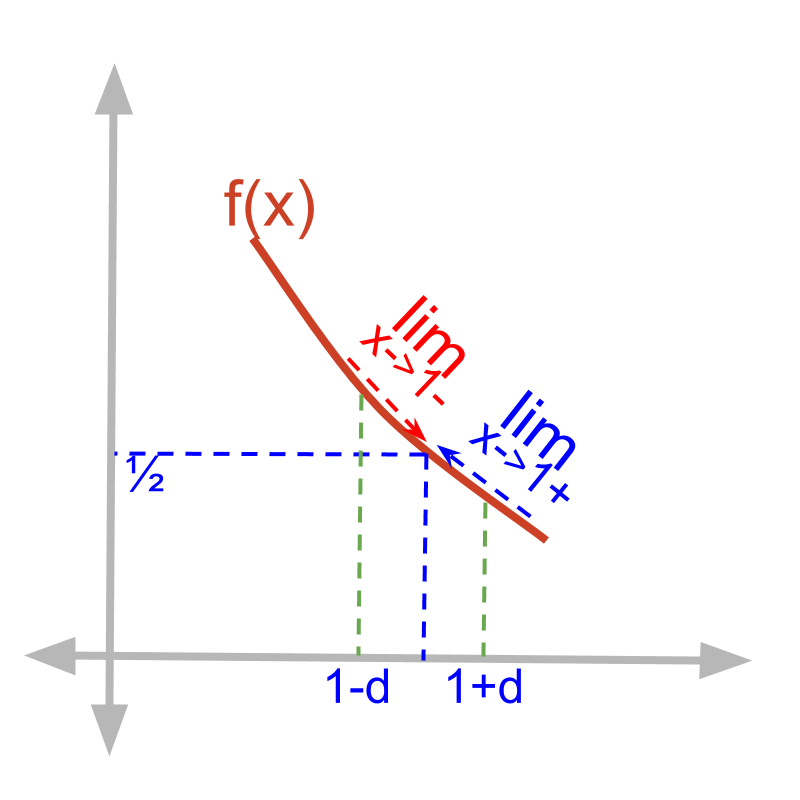→  continuous: if $f \left(a\right)$ = LHL = RHL

Limit of a defined Function

»  A function $f \left(x\right)$ at $x = a$ is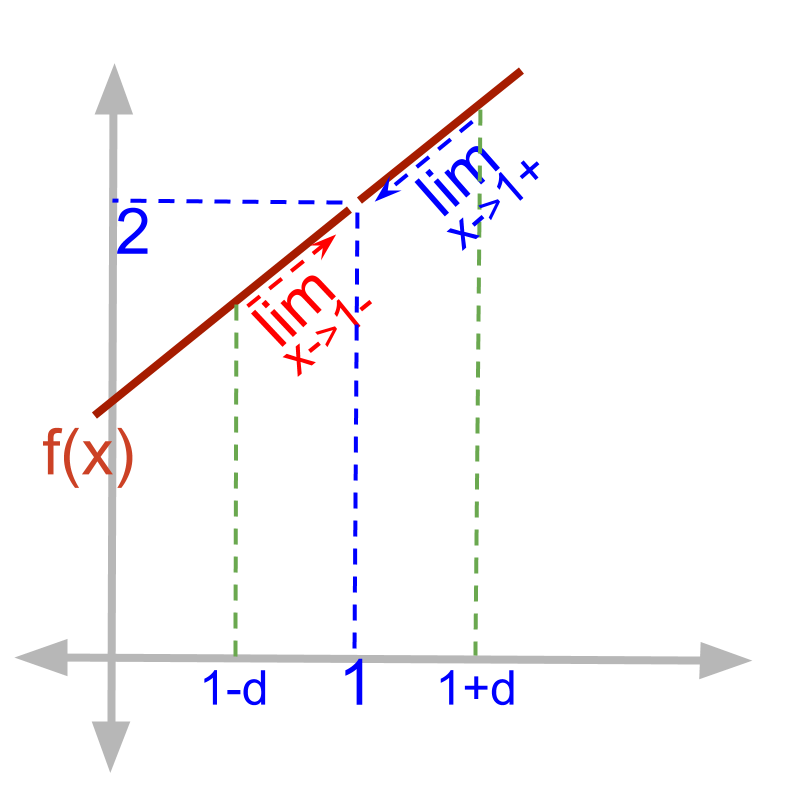→  defined by limit: if $f \left(a\right) = \frac{0}{0}$ and LHL = RHL

Limit of Piecewise function

»  A function $f \left(x\right)$ at $x = a$ is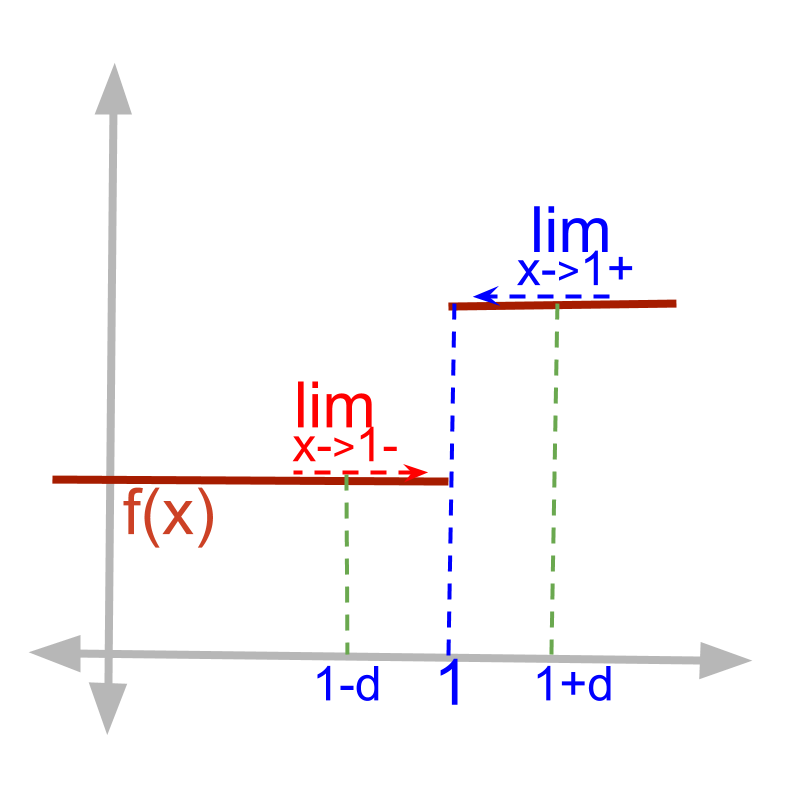→  not continuous and not defined: if LHL $\ne$ RHL

Limit of Function with abrupt change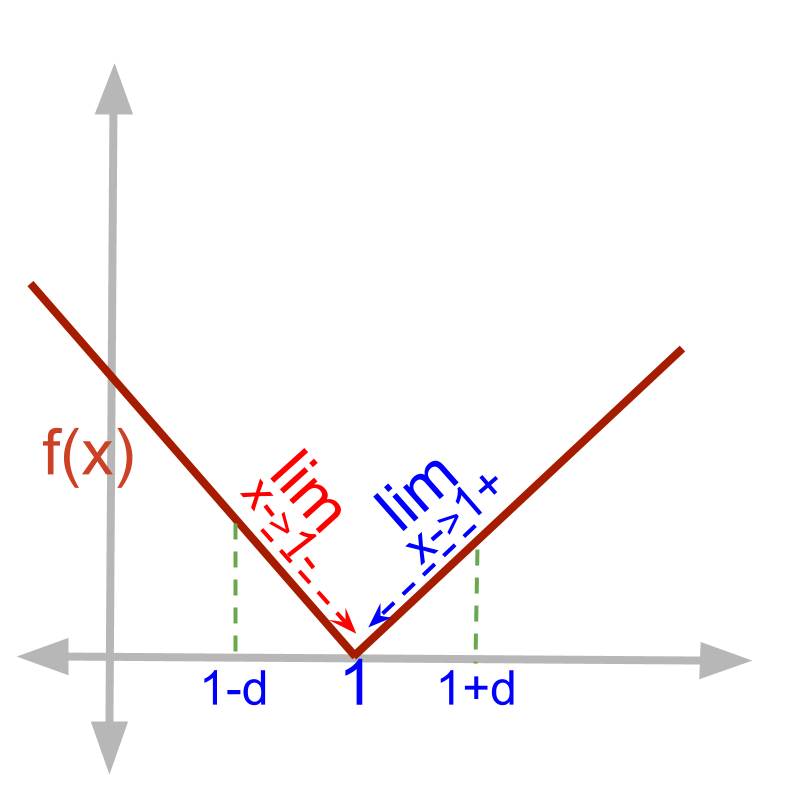»  A function $f \left(x\right)$ at $x = a$ is
→  continuous: if $f \left(a\right)$ = LHL = RHL

Limit of Functions that are not defined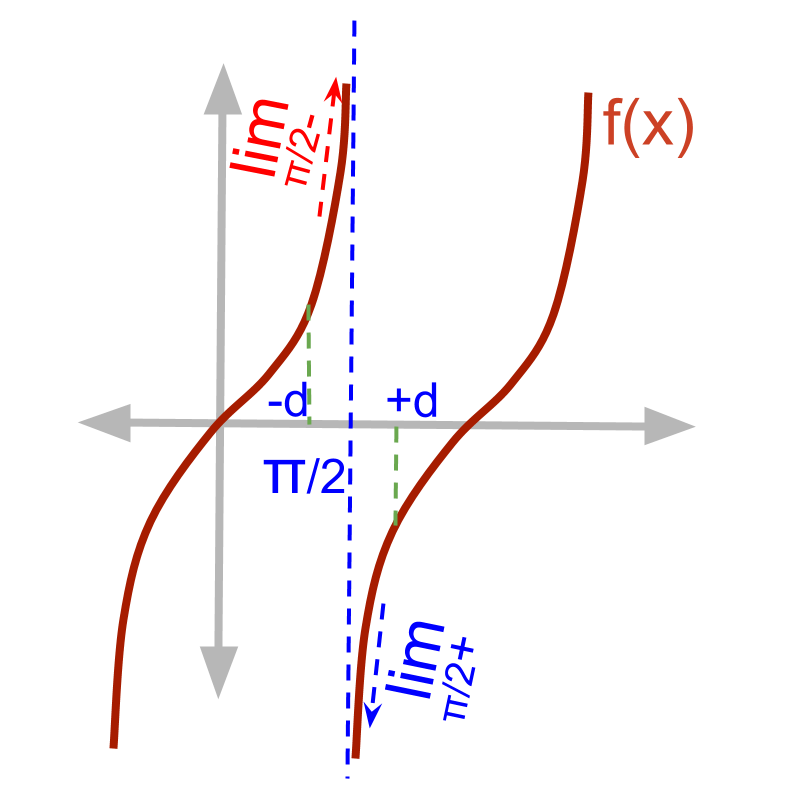»  A function $f \left(x\right)$ at $x = a$ is
→  not defined: if LHL $\ne$ RHL and $f \left(a\right) \notin \mathbb{R}$

check nature of function at a point

To decide if a function $f \left(x\right)$ is defined at an input value $x = a$, the following are examined.

$f \left(x\right) {|}_{x = a}$

${\lim}_{x \to a -} f \left(x\right)$

${\lim}_{x \to a +} f \left(x\right)$

It is important to note that the L'Hospital's rule is applicable only if the limit exists. The discussion in this topic is about finding if the limit exists or not. So the L'Hospital's rule is not used in finding the limits.

When a function is examined at an input value, the possible deductions one can make about the function at that input value are

Function is continuous.

Function is not continuous but defined.

Function is not continuous and not defined.

Whether a function is continuous or defined or not defined is determined by examining
• value at the input value,

• left-hand-limit at the input value, and

• right-hand-limit at the input value.

summary

Describing a function: A function $f \left(x\right)$ is evaluated for three values to describe at an input value $x = a$

•  $f \left(a\right)$

•  ${\lim}_{x \to a -} f \left(x\right)$

•  ${\lim}_{x \to a +} f \left(x\right)$

limit of continuous functions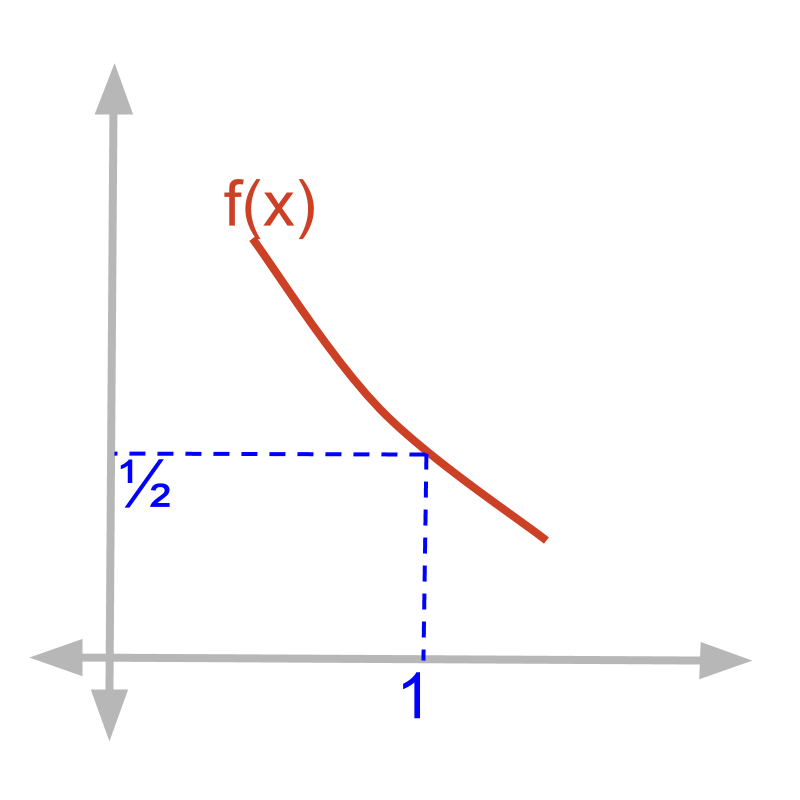Consider $f \left(x\right) = \frac{1}{{x}^{2} + 1}$

$f \left(x\right) {|}_{x = 1}$
Substitute $x = 1$
$= \frac{1}{1 + 1}$
$= \frac{1}{2}$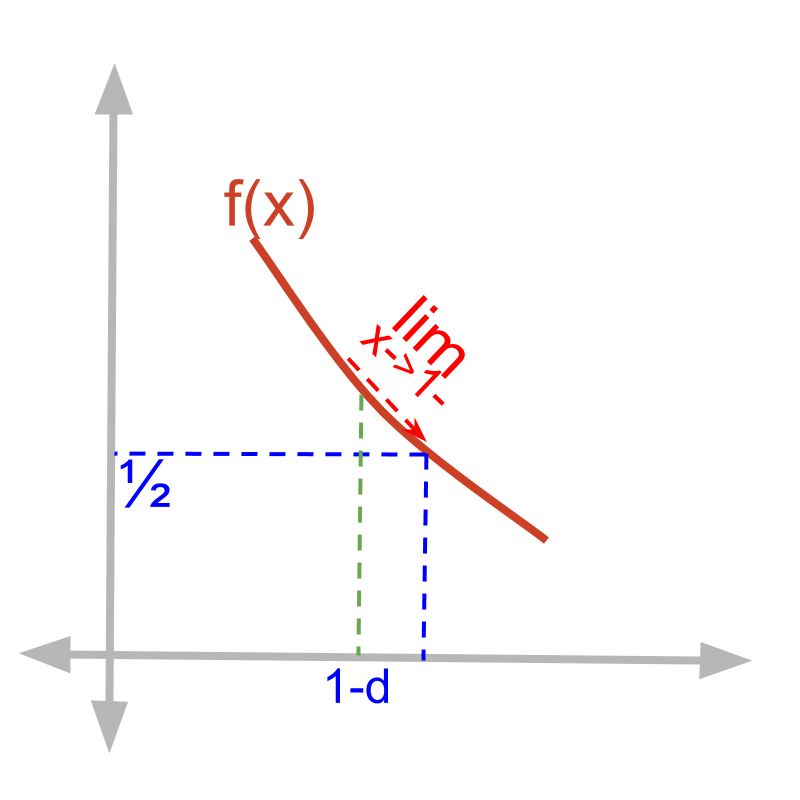Given function $f \left(x\right) = \frac{1}{{x}^{2} + 1}$.

The left-hand-limit ${\lim}_{x \to 1 -} f \left(x\right)$
$\quad \quad = \frac{1}{{\left(1 - \delta\right)}^{2} + 1}$
$\quad \quad = \frac{1}{1 - 2 \delta + {\delta}^{2} + 1}$
$\quad \quad = \frac{1}{2 - 2 \delta + {\delta}^{2}}$
$\quad \quad = \frac{1}{2} \quad \quad \quad \quad$ (substituting $\delta = 0$)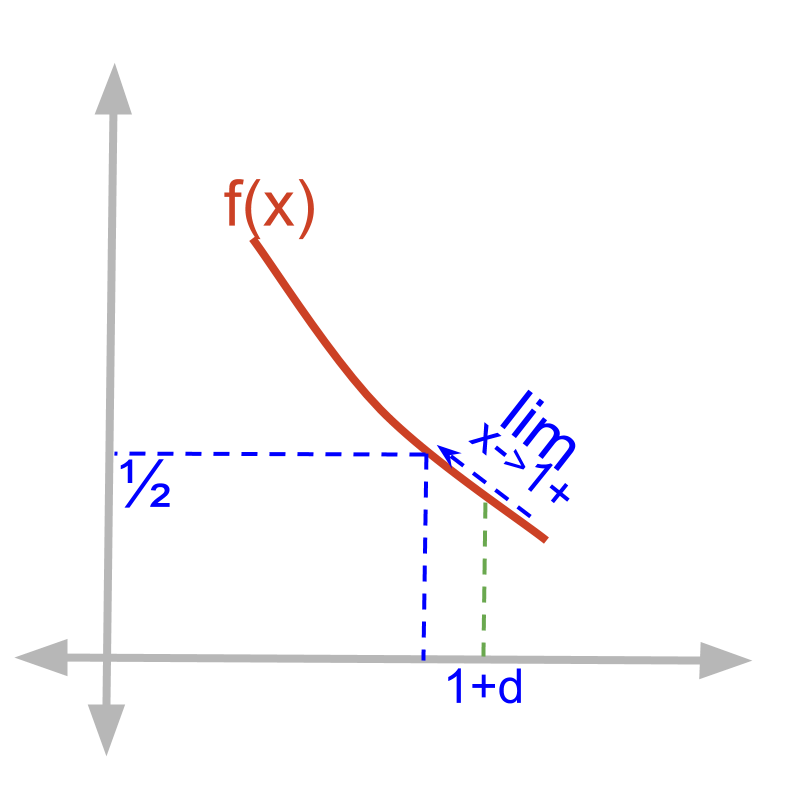Given function $f \left(x\right) = \frac{1}{{x}^{2} + 1}$.

The right-hand-limit ${\lim}_{x \to 1 +} f \left(x\right)$
$\quad \quad = \frac{1}{{\left(1 + \delta\right)}^{2} + 1}$
$\quad \quad = \frac{1}{1 + 2 \delta + {\delta}^{2} + 1}$
$\quad \quad = \frac{1}{2 + 2 \delta + {\delta}^{2}}$
$\quad \quad = \frac{1}{2} \quad \quad \quad \quad$ (substituting $\delta = 0$)Given function $f \left(x\right) = \frac{1}{{x}^{2} + 1}$.
•  $f \left(1\right) = \frac{1}{2}$
•  ${\lim}_{x \to 1 -} f \left(x\right) = \frac{1}{2}$
•  ${\lim}_{x \to 1 +} f \left(x\right) = \frac{1}{2}$

The function is continuous at $x = 1$.

Note: Though, as part of the topic, the functions that are discontinuous or indeterminate or non-differentiable are mainly handled, finding limits is not only for those kind of functions. Any function at any point can be evaluated for limits.

Function is continuous if all three values (1) function evaluated at the point, (2) left-hand-limit at the point and (3) right-hand-limit at the point are equal.

example

Given function $f \left(x\right) = \sin x$, is it continuous at input values $x = 0 , \frac{\pi}{2} , \pi$?

The answer is 'Continuous at all three input values'. This is a trivial problem as there is no

summary

Function is continuous: if $f \left(a\right)$ $= {\lim}_{x \to a -} f \left(x\right)$ $= {\lim}_{x \to a +} f \left(x\right)$, then the function is continuous at $x = a$.

Limit of defined functions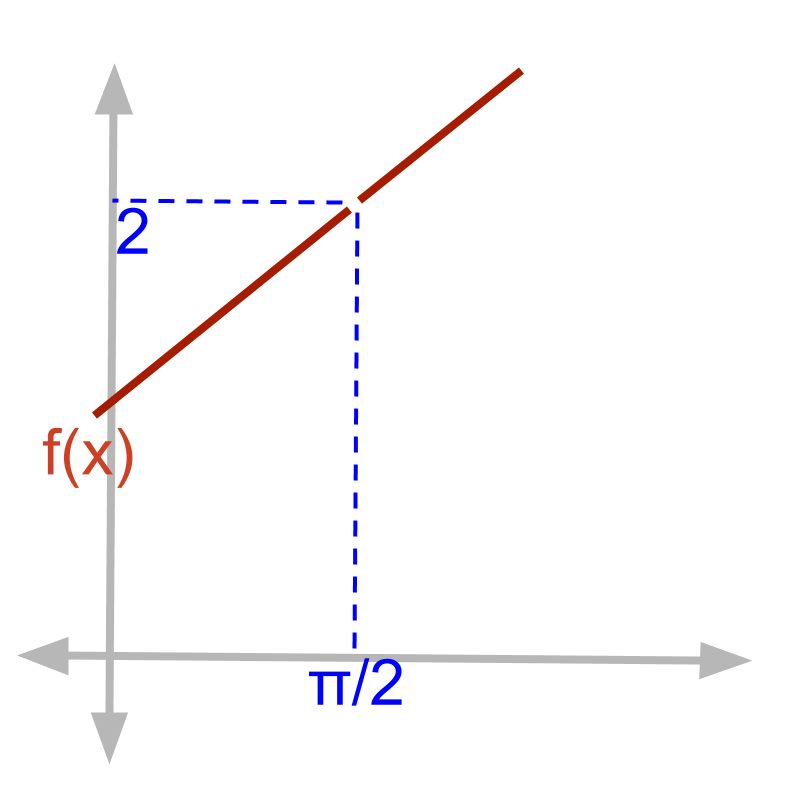Given function $f \left(x\right) = \frac{\textcolor{\mathrm{de} e p s k y b l u e}{{x}^{2} - 1}}{\textcolor{c \mathmr{and} a l}{x - 1}}$.

$f \left(x\right) {|}_{x = 1}$ Substituting $x = 1$ $= \frac{1 - 1}{1 - 1}$ $= \frac{0}{0}$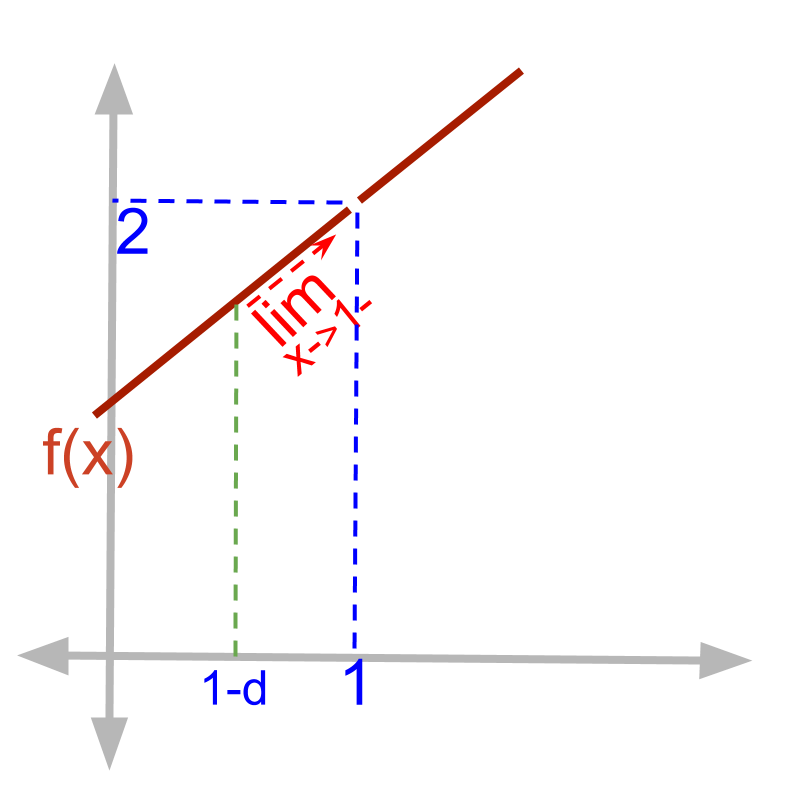Given function $f \left(x\right) = \frac{\textcolor{\mathrm{de} e p s k y b l u e}{{x}^{2} - 1}}{\textcolor{c \mathmr{and} a l}{x - 1}}$.

The left-hand-limit ${\lim}_{x \to 1 -} f \left(x\right)$
$\quad \quad = \frac{\textcolor{\mathrm{de} e p s k y b l u e}{{\left(1 - \delta\right)}^{2} - 1}}{\textcolor{c \mathmr{and} a l}{\left(1 - \delta\right) - 1}}$
$\quad \quad = \frac{\textcolor{\mathrm{de} e p s k y b l u e}{1 - 2 \delta + {\delta}^{2} - 1}}{\textcolor{c \mathmr{and} a l}{1 - \delta - 1}}$
$\quad \quad = \frac{\textcolor{\mathrm{de} e p s k y b l u e}{- \delta \left(2 - \delta\right)}}{\textcolor{c \mathmr{and} a l}{- \delta}}$
$\quad \quad = 2 - \delta$
$\quad \quad = 2$ (substituting $\delta = 0$)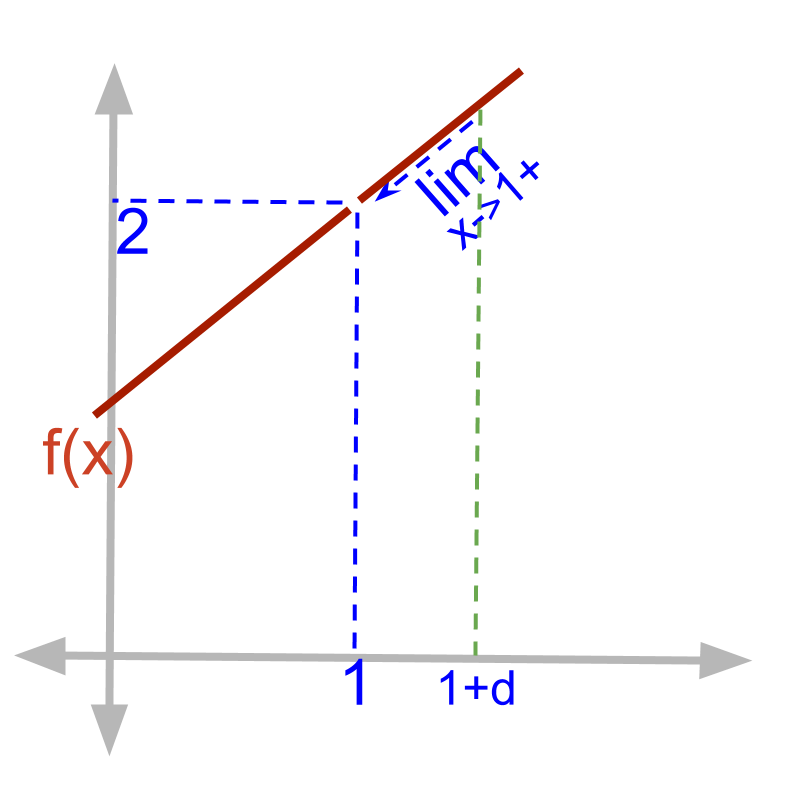Given function $f \left(x\right) = \frac{\textcolor{\mathrm{de} e p s k y b l u e}{{x}^{2} - 1}}{\textcolor{c \mathmr{and} a l}{x - 1}}$.

The right-hand-limit ${\lim}_{x \to 1 +} f \left(x\right)$
$\quad \quad = \frac{\textcolor{\mathrm{de} e p s k y b l u e}{{\left(1 + \delta\right)}^{2} - 1}}{\textcolor{c \mathmr{and} a l}{\left(1 + \delta\right) - 1}}$
$\quad \quad = \frac{\textcolor{\mathrm{de} e p s k y b l u e}{1 + 2 \delta + {\delta}^{2} - 1}}{\textcolor{c \mathmr{and} a l}{1 + \delta - 1}}$
$\quad \quad = \frac{\textcolor{\mathrm{de} e p s k y b l u e}{\delta \left(2 + \delta\right)}}{\textcolor{c \mathmr{and} a l}{\delta}}$
$\quad \quad = 2 + \delta$
$\quad \quad = 2$ (substituting $\delta = 0$)Given function $f \left(x\right) = \frac{\textcolor{\mathrm{de} e p s k y b l u e}{{x}^{2} - 1}}{\textcolor{c \mathmr{and} a l}{x - 1}}$.

•  $f \left(1\right) = \frac{0}{0}$ indeterminate value

•  ${\lim}_{x \to 1 -} f \left(x\right) = 2$

•  ${\lim}_{x \to 1 +} f \left(x\right) = 2$
The function is defined at $x = 1$.

When left-hand-limit and right-hand-limit are equal, then limit is defined. The two limits together are referred as "limit of the function".
That is, when it is mentioned / referred as "limit of a function", it means, both left and right hand limits are equal.

If the left hand and right hand limits are equal then the limit is defined at that input value.

If the function evaluates to indeterminate value and the limit is defined, then the function is defined by the limits.

example

Find if the function $f \left(x\right) = \frac{\textcolor{\mathrm{de} e p s k y b l u e}{3 {x}^{2} - 2 x - 8}}{\textcolor{c \mathmr{and} a l}{x - 2}}$ is continuous or defined at $x = 2$?

The answer is 'Not Continuous and Defined'

summary

Limit is defined: If ${\lim}_{x \to a -} f \left(x\right)$ $= {\lim}_{x \to a +} f \left(x\right)$ then the limits are commonly given as ${\lim}_{x \to a} f \left(x\right)$
Function is defined by Limit: If $f \left(a\right) \notin \mathbb{R}$ and ${\lim}_{x \to a -} f \left(x\right)$ $= {\lim}_{x \to a +} f \left(x\right)$, then the function is defined by limit at $x = a$.

Limit of piecewise functions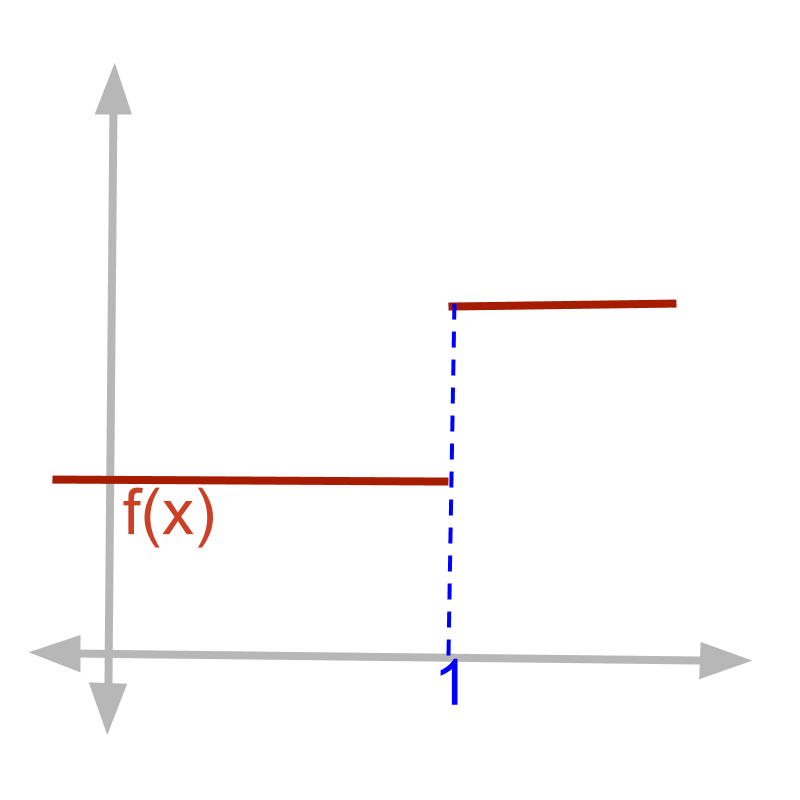Given piecewise function
$f \left(x\right) = \left\{\begin{matrix}1 & \quad \textrm{f \mathmr{and}} \quad x \ge 1 \\ 0.5 & \quad \textrm{f \mathmr{and}} \quad x < 1\end{matrix}\right.$

$f \left(x\right) {|}_{x = 1} = 1$ as given by definition of the function.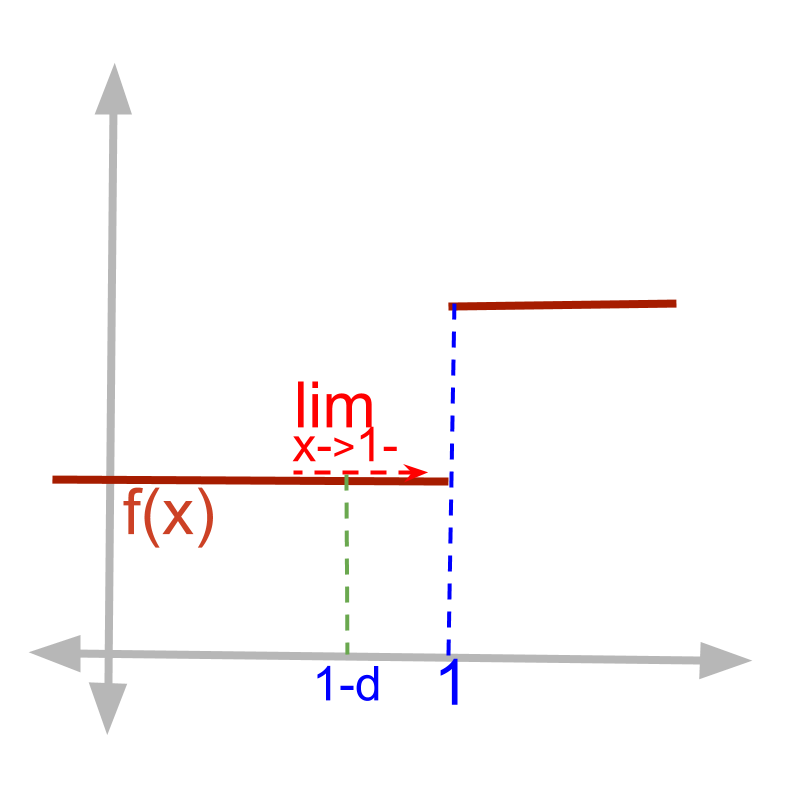Given function
$f \left(x\right) = \left\{\begin{matrix}1 & \quad \textrm{f \mathmr{and}} \quad x \ge 1 \\ 0.5 & \quad \textrm{f \mathmr{and}} \quad x < 1\end{matrix}\right.$

The left-hand-limit ${\lim}_{x \to 1 -} f \left(x\right)$
$\quad \quad = f \left(x\right) {|}_{x = 1 - \delta}$
$\quad \quad = 0.5 \quad \quad \quad \quad$ (as $1 - \delta < 1$)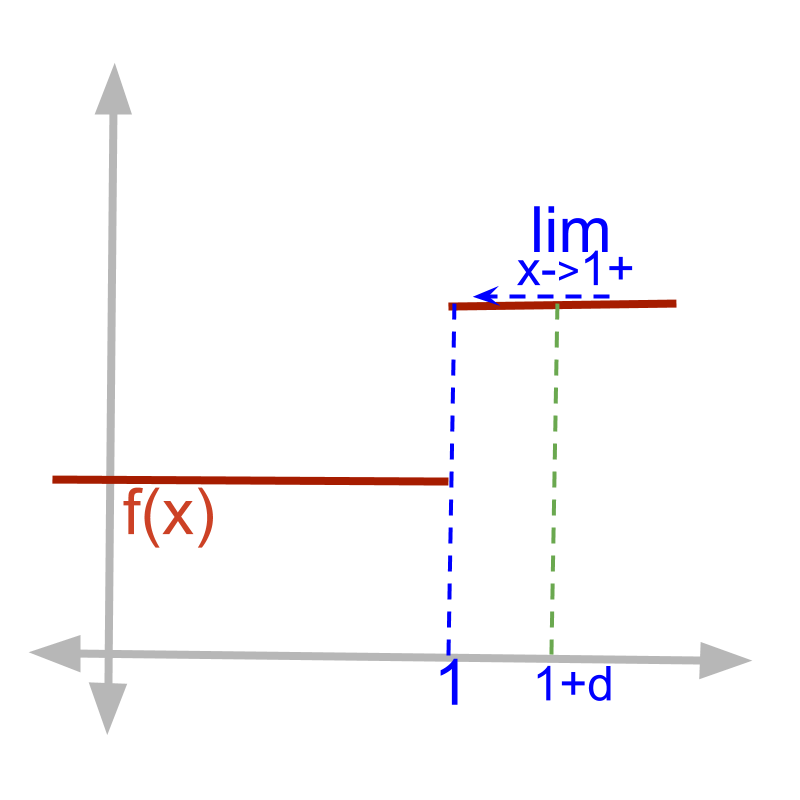Given function
$f \left(x\right) = \left\{\begin{matrix}1 & \quad \textrm{f \mathmr{and}} \quad x \ge 1 \\ 0.5 & \quad \textrm{f \mathmr{and}} \quad x < 1\end{matrix}\right.$

The right-hand-limit ${\lim}_{x \to 1 +} f \left(x\right)$
$\quad \quad = f \left(x\right) {|}_{x = 1 + \delta}$
$\quad \quad = 1 \quad \quad \quad \quad$ (as $1 + \delta > 1$)Given function
$f \left(x\right) = \left\{\begin{matrix}1 & \quad \textrm{f \mathmr{and}} \quad x \ge 1 \\ 0.5 & \quad \textrm{f \mathmr{and}} \quad x < 1\end{matrix}\right.$

•  $f \left(1\right) = 1$

•  ${\lim}_{x \to 1 -} f \left(x\right) = 0.5$

•  ${\lim}_{x \to 1 +} f \left(x\right) = 1$

The function is not continuous at $x = 1$. But, the function is defined by the given definition of the function.

If the function evaluates to a real number, then the function is defined at that input value. The limit need not be defined.

example

Given function
$f \left(x\right) = \left\{\begin{matrix}2 x & \quad \textrm{f \mathmr{and}} \quad x > 2 \\ {x}^{2} & \quad \textrm{f \mathmr{and}} \quad x = 2 \\ | x | + 2 & \quad \textrm{f \mathmr{and}} \quad x < 2\end{matrix}\right.$
Examine the function at $x = 2$.

The answer is 'All the above'.

summary

Function is defined by value: If $f \left(a\right) \in \mathbb{R}$, and ${\lim}_{x \to a -} f \left(x\right)$ $\ne {\lim}_{x \to a +} f \left(x\right)$, then function is not continuous but defined by the definition of the function.

Limit of functions with abrupt change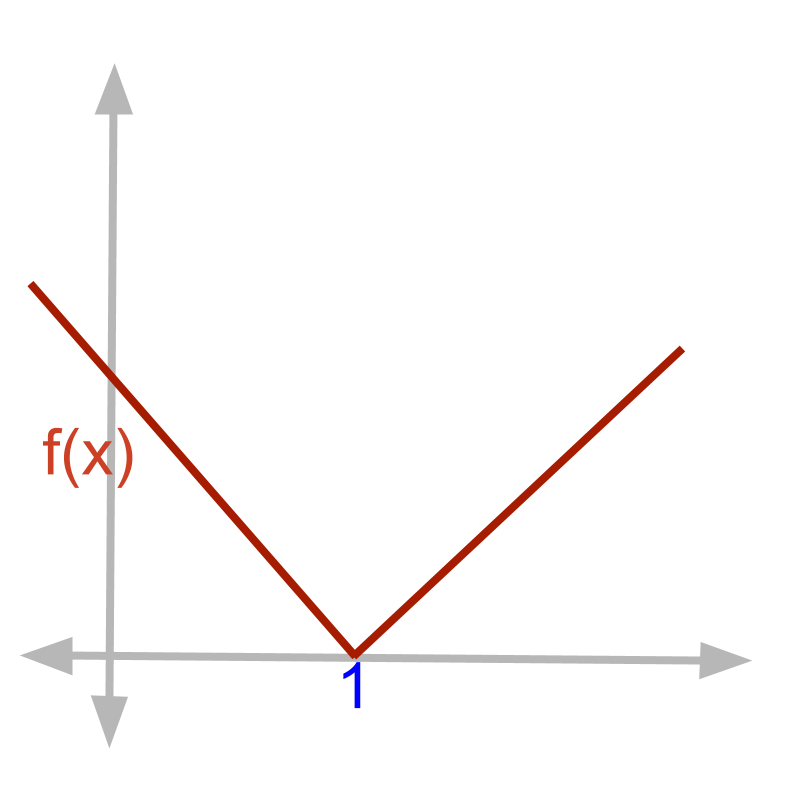Given function $f \left(x\right) = | x - 1 |$.

$f \left(x\right) {|}_{x = 1}$
$= | 1 - 1 |$
$= 0$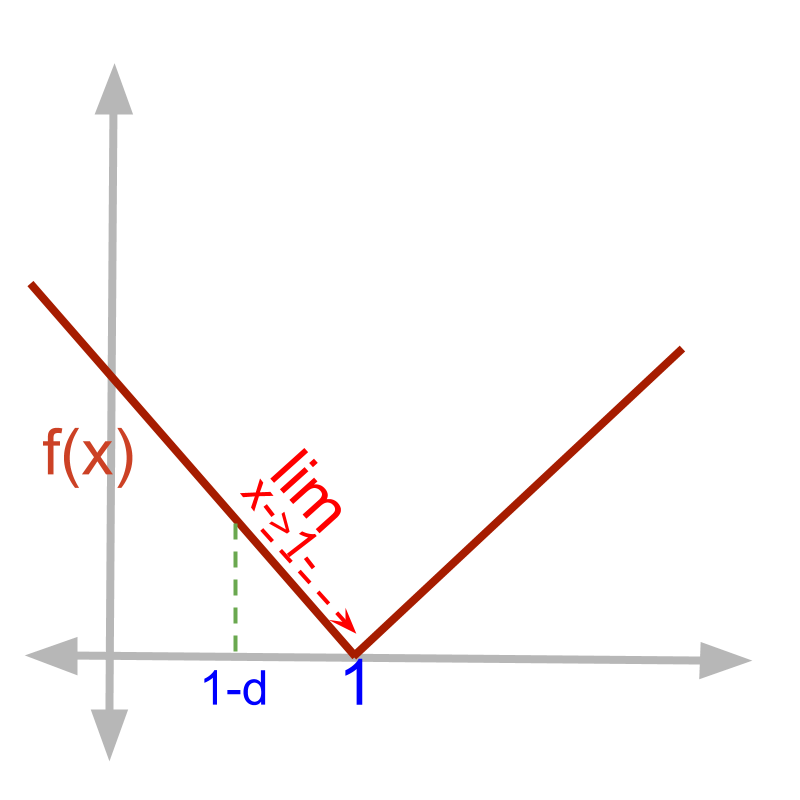Given function $f \left(x\right) = | x - 1 |$.

The left-hand-limit ${\lim}_{x \to 1 -} f \left(x\right)$
$\quad \quad = | 1 - \delta - 1 |$
$\quad \quad = | - \delta |$
$\quad \quad = \delta$
$\quad \quad = 0 \quad \quad \quad \quad$ substituting $\delta = 0$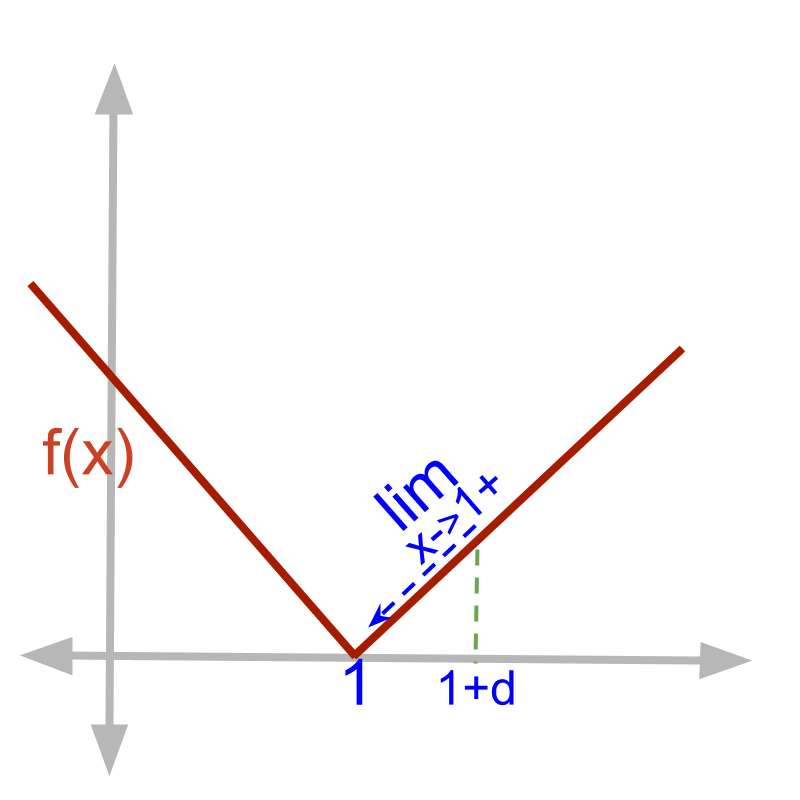Given function $f \left(x\right) = | x - 1 |$.

The right-hand-limit ${\lim}_{x \to 1 +} f \left(x\right)$
$\quad \quad = | 1 + \delta - 1 |$
$\quad \quad = | \delta |$
$\quad \quad = \delta$
$\quad \quad = 0 \quad \quad \quad \quad$ substituting $\delta = 0$Given function $f \left(x\right) = | x - 1 |$.

•  $f \left(1\right) = 0$

•  ${\lim}_{x \to 1 -} f \left(x\right) = 0$

•  ${\lim}_{x \to 1 +} f \left(x\right) = 0$

The function is continuous at $x = 1$. Note that the function undergoes an abrupt change at $x = 1$.

•  for $x < 1$, the slope of the line is $- 1$

•  for $x > 1$, the slope of the line is $1$

•  At $x = 1$, the slope of the line is not defined.

example

Given the function $f \left(x\right) = | \sin x |$. Is the function continuous or defined at $x = \pi$?

Limit of Functions that are not defined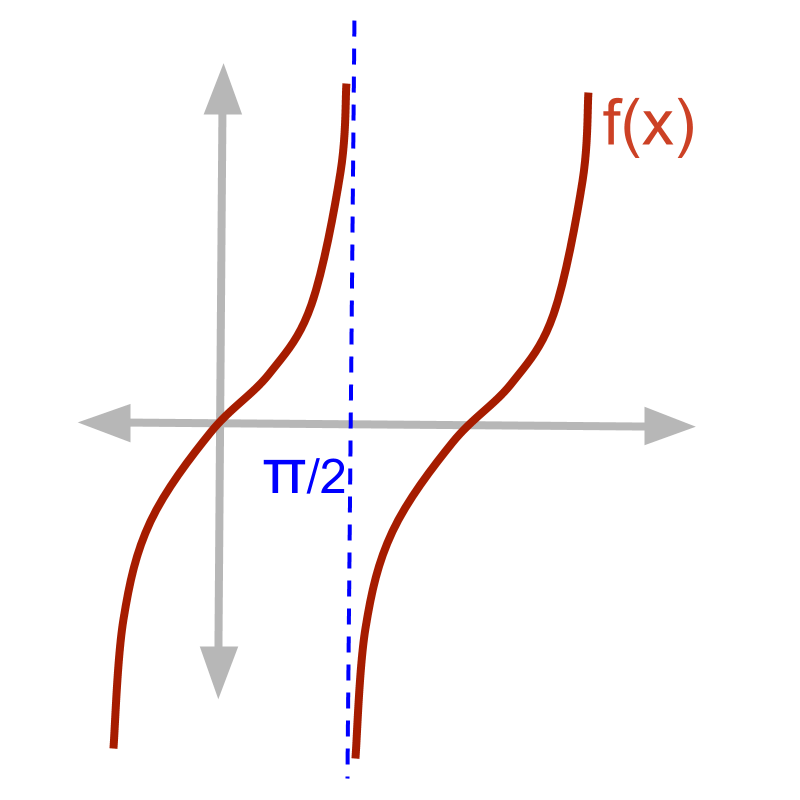Given function $f \left(x\right) = \tan x$.

$f \left(x\right) {|}_{x = \frac{\pi}{2}}$
$\quad \quad = \tan \left(\frac{\pi}{2}\right)$
$\quad \quad = \infty$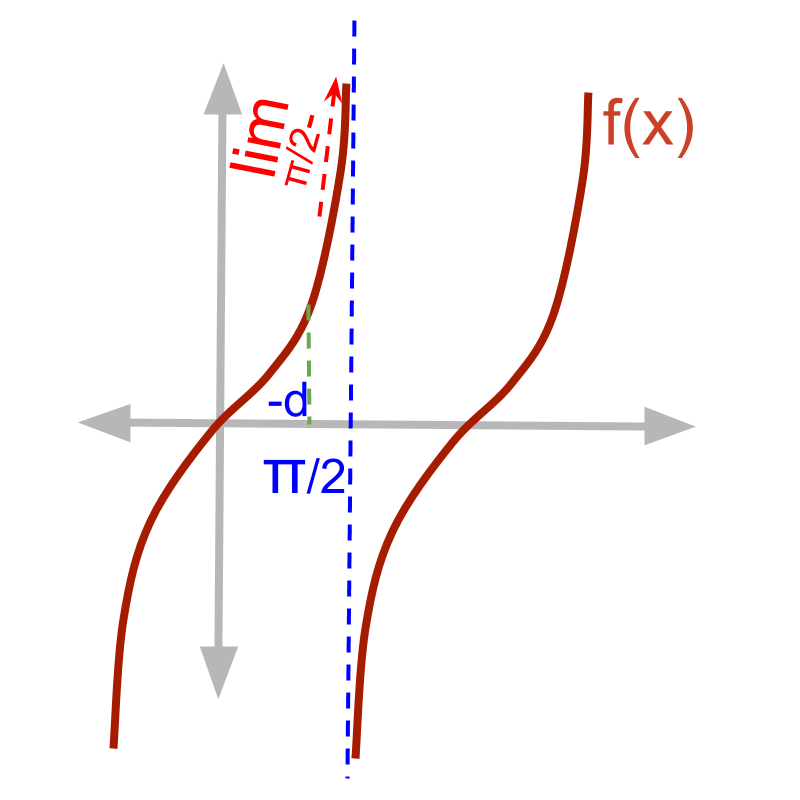Given function $f \left(x\right) = \tan x$.

The left-hand-limit ${\lim}_{x \to \frac{\pi}{2} -} f \left(x\right)$
$\quad \quad = f \left(\frac{\pi}{2} - \delta\right)$
$\quad \quad = \tan \left(\frac{\pi}{2} - \delta\right)$
$\quad \quad = \frac{\sin \left(\frac{\pi}{2} - \delta\right)}{\cos \left(\frac{\pi}{2} - \delta\right)}$
$\quad \quad = \frac{\cos \left(\delta\right)}{\sin \left(\delta\right)}$
$\quad \quad = \frac{1}{0}$
$\quad \quad = \infty$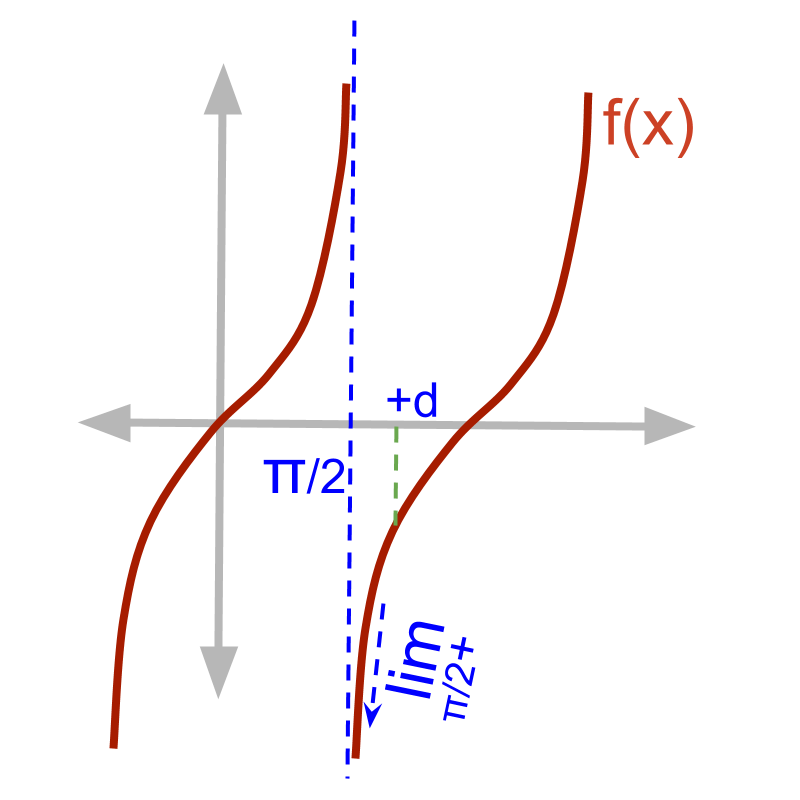Given function $f \left(x\right) = \tan x$.

The right-hand-limit ${\lim}_{x \to \frac{\pi}{2} +} f \left(x\right)$
$\quad \quad = f \left(\frac{\pi}{2} + \delta\right)$
$\quad \quad = \tan \left(\frac{\pi}{2} + \delta\right)$
$\quad \quad = \frac{\sin \left(\frac{\pi}{2} + \delta\right)}{\cos \left(\frac{\pi}{2} + \delta\right)}$
$\quad \quad = \frac{\cos \left(\delta\right)}{- \sin \left(\delta\right)}$
$\quad \quad = - \frac{1}{0}$
$\quad \quad = - \infty$Given function $f \left(x\right) = \tan x$.

•  $f \left(\frac{\pi}{2}\right) = \infty$

•  ${\lim}_{x \to \frac{\pi}{2} -} f \left(x\right) = \infty$

•  ${\lim}_{x \to \frac{\pi}{2} +} f \left(x\right) = - \infty$
The function is not continuous and not defined at $x = \frac{\pi}{2}$.

If the function evaluates to $\pm \infty$ or $\frac{0}{0}$ and the limits are not equal, then the function is not defined at that input value.

If a function does not have value in real numbers and the two limits are not equal then the function is not defined at that input value.

example

Examine the function $f \left(x\right) = \frac{1}{x - 3}$ at $x = 3$.

Function not Defined: If $f \left(a\right) \notin \mathbb{R}$ and ${\lim}_{x \to a -} f \left(x\right)$ $\ne {\lim}_{x \to a +} f \left(x\right)$ then the function is not defined at $x = a$.